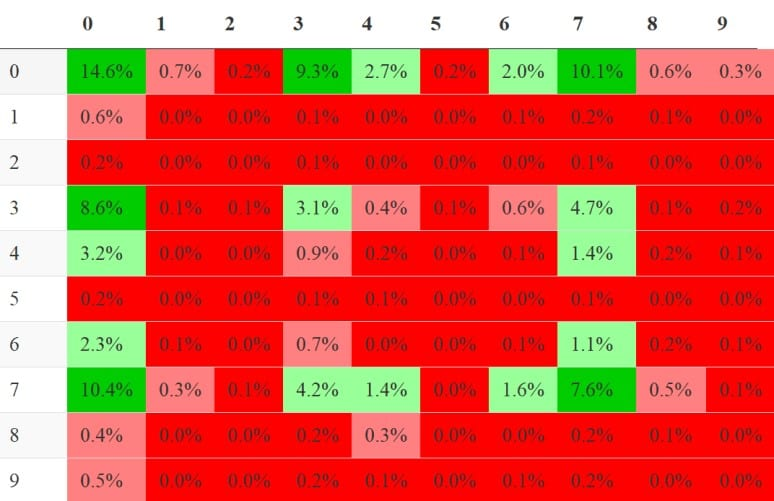Have you ever heard someone ask the question, “What’s the odds of that happening?”. Well, that’s the concept of probability. Let’s learn a little more about probability and how it can be used in your organization.

When you start discussing probability with someone else in your organization, you may hear the words deterministic or stochastic. If something is referred to as deterministic, then its outcome is certain and known with a probability of 1. If something is stochastic then its outcomes are a function of random probability.

## Overview: What is probability?

Probability is a measure of the likelihood of an event occurring. It is expressed as a number between 0 and 1, where 0 represents an impossible event and 1 represents a certain event. A probability of 0.5, for example, indicates that an event is equally likely to occur or not occur. In probability theory, probabilities are used to model uncertain events and make predictions about the outcomes of random processes.

Probability is the assignment of a value between zero and one for every event. The probability of an event A is written as P(A). For event B it would be P(B). If two events A and B can occur together, this is called the joint probability of A and B, denoted as P(A∩B).

If either event A or event B can occur but never both simultaneously, then they are called mutually exclusive events. If two events are mutually exclusive, then the probability of both occurring is denoted as P(A∩B) and P(A and B). If two events are mutually exclusive, then the probability of either occurring is denoted as P(A∪B) and P(A or B).

Conditional probability is the probability of some event A, given the occurrence of some other event B. Conditional probability is written as P(A∣B) and is read “the probability of A, given B“. In all cases, the sum of all probabilities for a set of outcomes must total 1 or 100%.

## An industry example of probability

At the company Super Bowl party, the participants decided to create a Super Bowl Squares Matrix. Super Bowl Squares is a game where people enter into a pool and are assigned a pair of two single-digit numbers like “7/6”, or “3/3”, or “1/2” etc. These numbers represent the one’s digit of the scores for the home team and the away team. For example, if the home team is winning 12-10 at the end of the first quarter, whoever has the number pairing “2/0” wins a portion of the pot. Awards are paid out at the end of the first, second, and third quarters as well as at the end of the game.

The company’s Six Sigma Master Black Belt (MBB) was pretty good at statistics so she gathered past data from hundreds of past football games and calculated the probabilities of the different scoring combinations. Since she could only stay until the 3rd quarter, she developed the probability matrix below to help her determine which scores to bet on.Probabilities for Super Bowl Squares

As you can see, the highest probabilities of winning are with the 0/0, 7/0, 0/7 and the 0/3 and 3/0 squares. Unfortunately, this was a stochastic event and none of her selections occurred.

### What is a simple definition of probability?

Simply speaking, probability is the likelihood or chance of an event occurring. The probability of this event will be between 0 (uncertainty) and 1 (certainty). Probability can be expressed in terms of decimals or percentages.

### What is conditional probability?

It is the likelihood or probability of an event occurring based on the occurrence of a prior event or outcome.

### What is the probability of a deterministic event?

A deterministic event is one with a known probability and is free of random variation. Therefore, it is certain and thus has a probability of 1 or 100%.